# 小白给小白详解维特比算法（二）

https://blog.csdn.net/athemeroy/article/details/79342048

P（身体状态=正常）=0.7
P（身体状态=轻感冒）=0.2
P（身体状态=重感冒）=0.1

• 1
• 2
• 3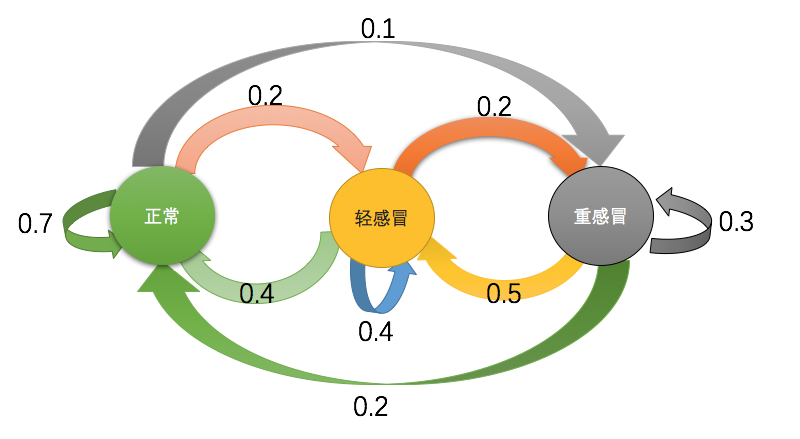Emmmm…看上去有点乱，如果只有这么三个状态还好，如果状态再多点，感觉就要眼花缭乱了！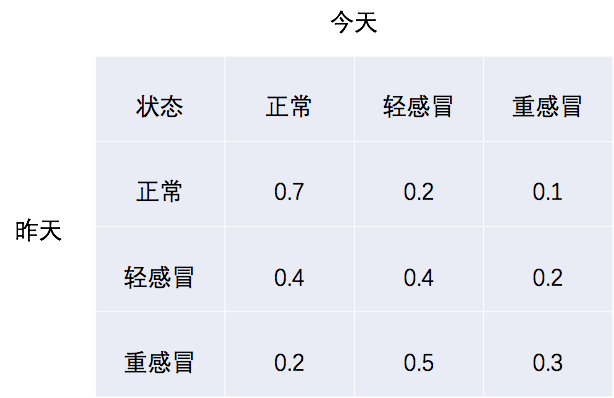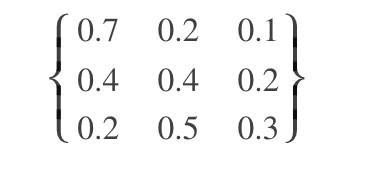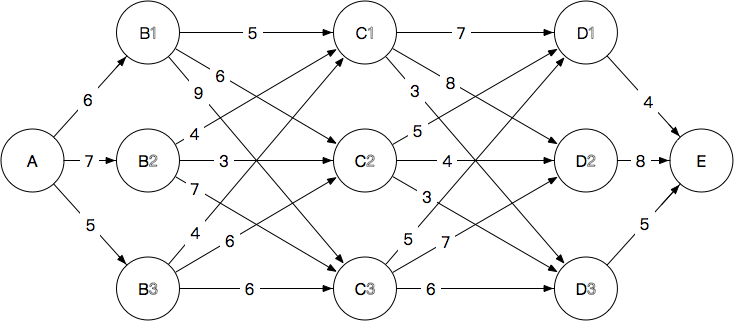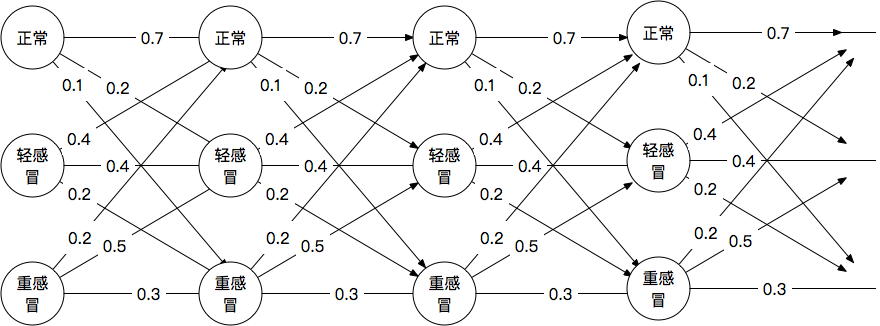“我能在河边，画上一整天的图！”纳特·帕格兴高采烈地说道。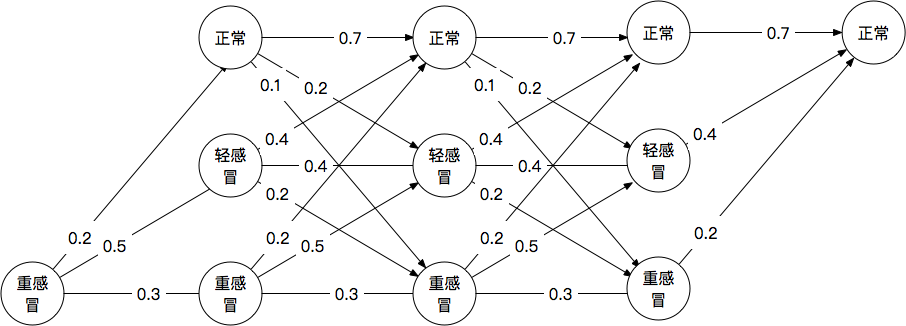注：为什么我们简单把路径上的概率乘在一起就可以呢？（一阶）马尔科夫假设事实上是这样一个东西（竖线|表示“给定，以…为条件”）：image_1c6qsenns13e97qfd7k10bl1b6m9.png-1.9kB（来自维基百科），即在已经出现了前N个状态的条件下得到第N+1个状态的概率等于已经出现了第N个状态时出现第N+1个概率（所以才说“和前天无关只和昨天有关”）。而根据条件概率，我们有
P(Xn+1=轻感冒,Xn=重感冒)=P(Xn+1=轻感冒|Xn=重感冒)P(Xn=重感冒)(0)

P(Xn+2=正常,Xn+1=轻感冒,Xn=重感冒)=(1)
P(Xn+2=重感冒|Xn+1=轻感冒,Xn=重感冒)×P(Xn+1=轻感冒,Xn=重感冒)

P(Xn+2=正常|Xn+1=轻感冒,Xn=重感冒)=P(Xn+2=正常|Xn+1=轻感冒)

P(Xn+2=正常,Xn+1=轻感冒,Xn=重感冒)=
P(Xn+2=正常|Xn+1=轻感冒)×P(Xn+1=轻感冒,Xn=重感冒)

P(Xn+1=轻感冒,Xn=重感冒)=P(Xn+1=轻感冒|Xn=重感冒)P(Xn=重感冒)

P(Xn+2=正常,Xn+1=轻感冒,Xn=重感冒)=
P(Xn+2=正常|Xn+1=轻感冒)P(Xn+1=轻感冒|Xn=重感冒)P(Xn=重感冒)


• 1
• 2
• 3
• 4
• 5
• 6
• 7
• 8
• 9
• 10
• 11
• 12
• 13
• 14
• 15
• 16
• 17
• 18

## 什么是隐马尔科夫模型？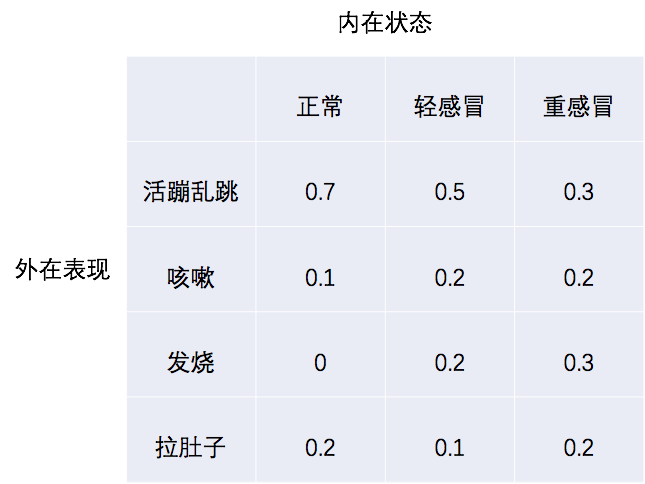## 隐马尔科夫模型与篱笆网络

已知某人第一天轻感冒，第二天发烧，求第二天最可能的内在状态。

• 1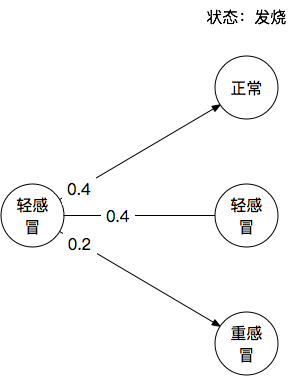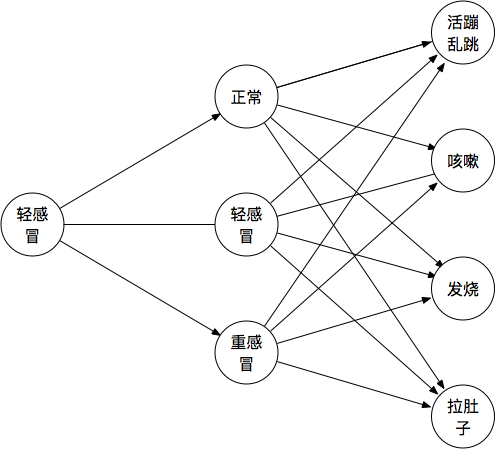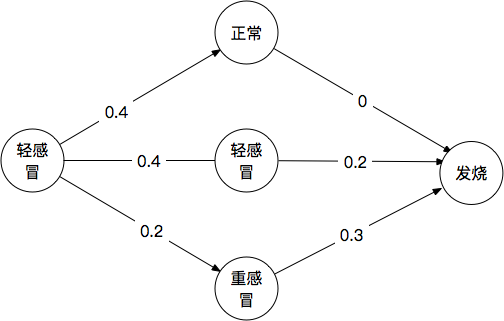=P(Yn=发烧|Xn−1=轻感冒,Xn=轻感冒)P(Xn−1=轻感冒,Xn=轻感冒) 而因为独立输出假设，第n天外在表现为“发烧”，仅仅和第n天内在的状态有关，和前一天的状态无关：P(Yn=发烧|Xn−1=轻感冒,Xn=轻感冒)=P(Yn=发烧|Xn=轻感冒)

=P(Yn=发烧|Xn=轻感冒)P(Xn−1=轻感冒,Xn=轻感冒)其中后一项我们上文已经讨论过很多次了，通过转移概率很容易计算，而前一项就是内在状态到外在表现的发射概率。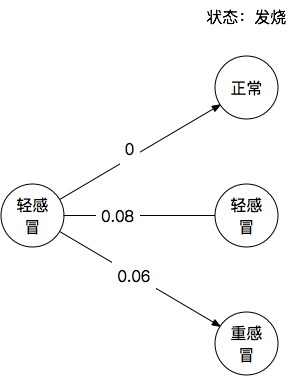P(Xn=轻感冒|Yn=发烧,Xn−1=轻感冒)而不单单是联合概率。根据贝叶斯公式我们有 P(Xn=轻感冒|Yn=发烧,Xn−1=轻感冒)

=P(Xn−1=轻感冒,Xn=轻感冒,Yn=发烧)P(Yn=发烧,Xn−1=轻感冒)
=P(Yn=发烧|Xn−1=轻感冒,Xn=轻感冒)P(Xn−1=轻感冒,Xn=轻感冒)P(Yn=发烧,Xn−1=轻感冒)
=P(Yn=发烧|Xn=轻感冒)P(Xn−1=轻感冒,Xn=轻感冒)P(Yn=发烧,Xn−1=轻感冒) 而分数线下面的概率（归一化系数），输出仅与当天的内在状态有关，和前一天的状态无关
=P(Yn=发烧|Xn=轻感冒)P(Xn−1=轻感冒,Xn=轻感冒)P(Yn=发烧)P(Xn−1=轻感冒) 根据全概率公式
=P(Yn=发烧|Xn=轻感冒)P(Xn−1=轻感冒,Xn=轻感冒)∑XnP(Yn=发烧|Xn)P(Xn−1=轻感冒) 看得出这个归一化系数就是各条路径的和，它总是个常数。所以如果我们仅仅考虑哪条路径最大，是否归一化其实也区别不大。

• n-gram语言模型
• 不是题外话的题外话：拼音输入法
• 标注问题
维特比算法怎么解决标注问题？
posted @ 2019-06-28 12:44  交流_QQ_2240410488  阅读(109)  评论(0编辑  收藏